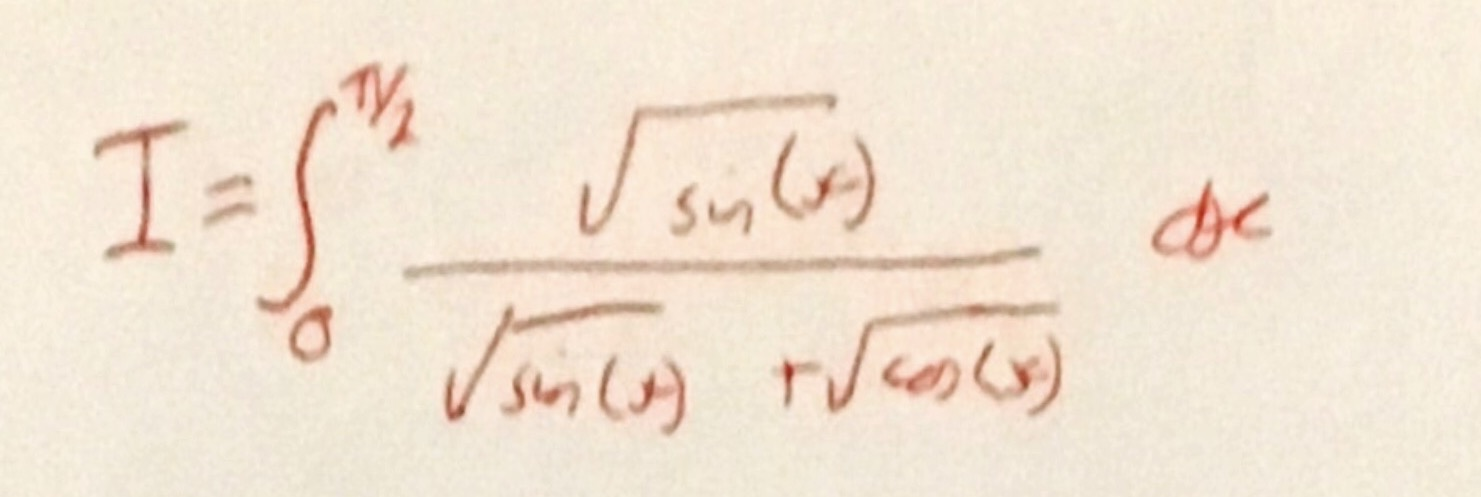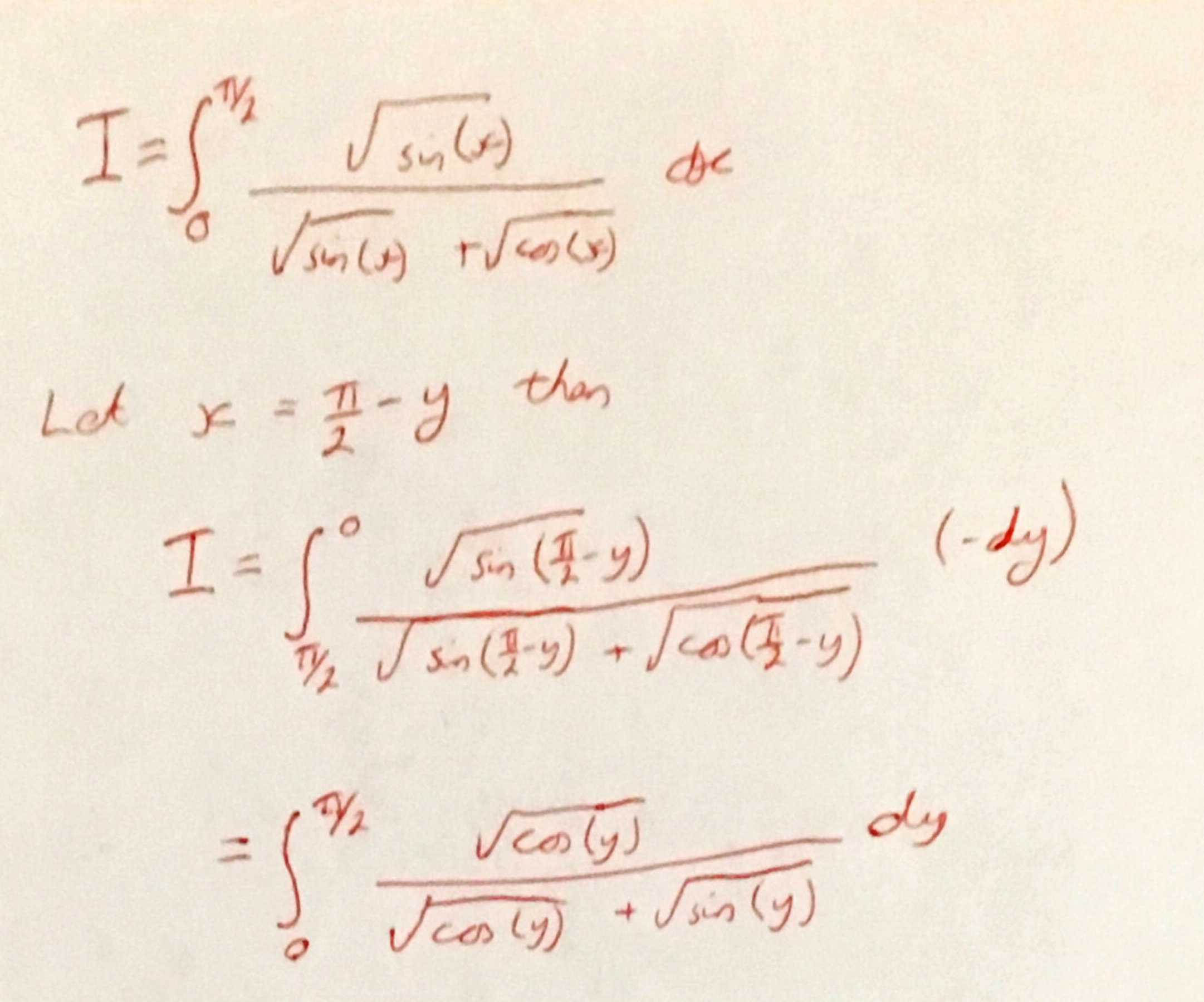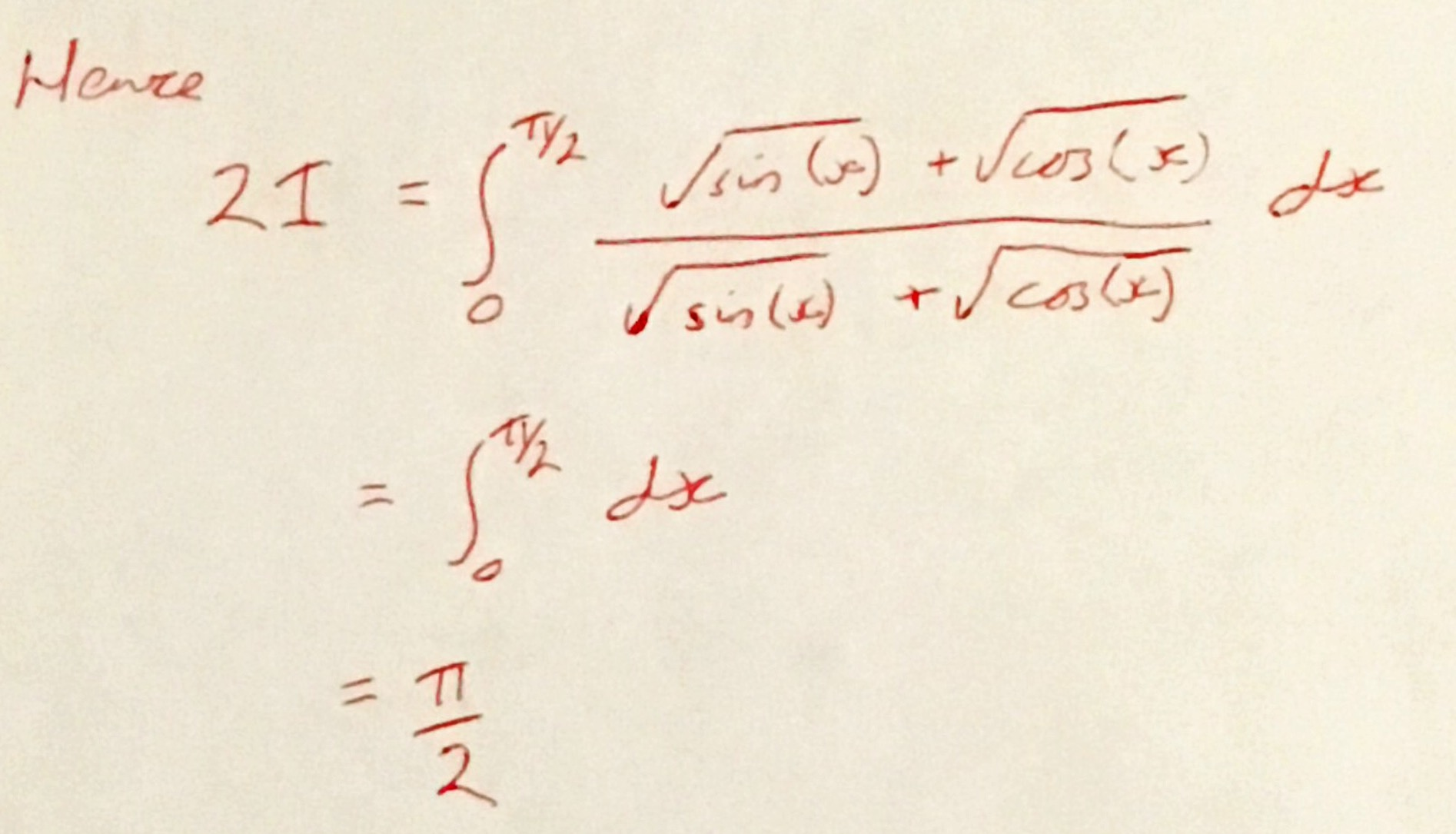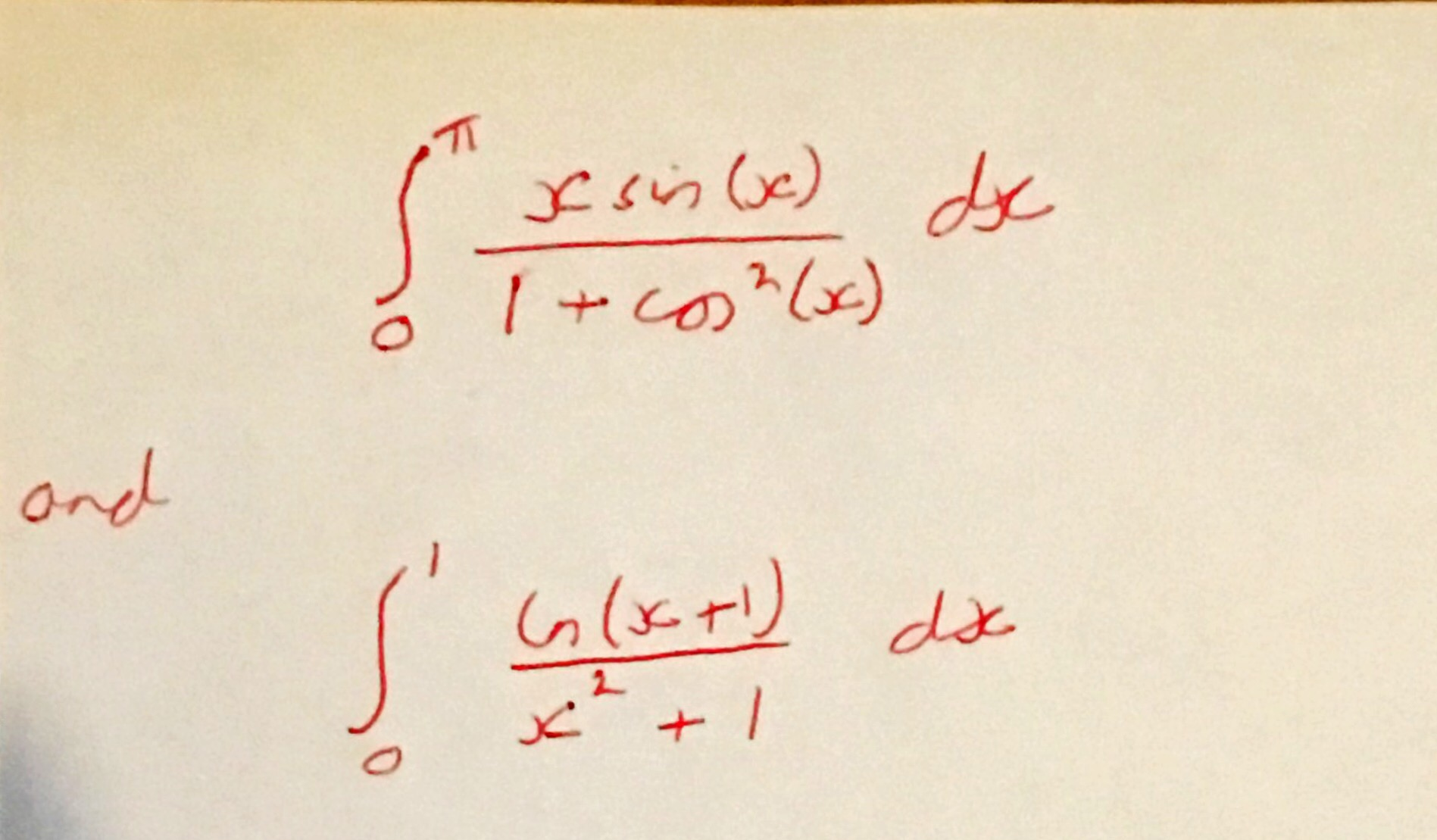# An Integration Trick

Before I started teaching A-Level I hadn’t done an integral by hand for about 5 years – I would always use Mathematica when I had to evaluate an integral symbolically.

I’ve actually enjoyed re-familiarising myself with some of the techniques required for some of the more difficult integrands and fancied looking at some more challenging integrals and so I bought the book “Inside Interesting Integrals” by Paul J. Nahin. This is a great book packed full of clever tricks to evaluate integrals and I thought as it is the last day of the holidays (and so don’t want to write a long post) I would share one of them here.

It was nice to be reminded of the “flipping the integral’s variable’s direction” trick which works in a lot of places. For example consider the integral below.Make the substitution $$x = \frac{\pi}{2}-y$$ and so $$dx = -dy$$ which gives the followingand so, by adding this expression to the original integral (and changing back the dummy variable of integration) you obtainHence, the original definite integral is equal to $$\pi / 4$$. I think this is pretty neat!

Here are some for you to try (you may need to pick a substitution as well as using the trick described above):